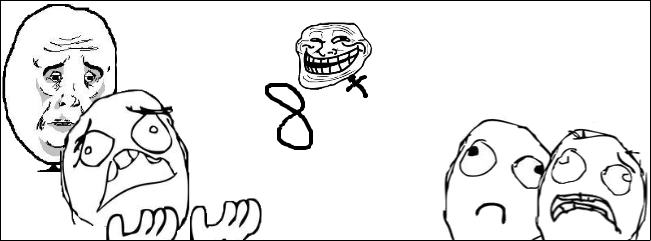# $x$ in the Exponents? Not again!

Algebra Level 2The equation $\dfrac{8^x+27^x}{12^x+18^x}=\dfrac76$ has two integer solutions, we'll denote as $a,b$. Find the value of $\sqrt{\color{#FFFFFF}{\overline{{\color{#333333}{2a^2+2b^2}}}}}.$

×